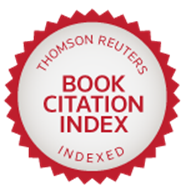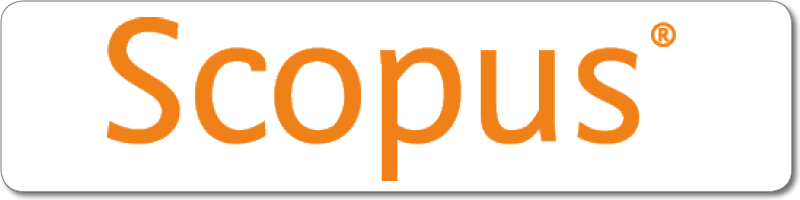| |
River Publishers Series in Mathematical, Statistical and Computational Modelling for Engineering

## Textbook on Ordinary Differential Equations Forthcoming

#### A Theoretical Approach

Author: Ramakanta Meher, Sardar Vallabhbhai National Institute of Technology, India

ISBN: 9788770227636
e-ISBN: 9788770227629

Price: \$ 126.50Distributed exclusively by Routledge (Taylor & Francis Group)Description:

Many scientific and real-world problems that occur in science, engineering, and medicine can be represented in differential equations. There is a vital role for differential equations in studying the behavior of different types of real-world problems. Thus, it becomes crucial to know the existence uniqueness properties of differential equations and various methods of finding differential equation solutions in explicit form. It is also essential to know different kinds of differential equations in terms of eigenvalues, termed eigenvalue problems, and some special functions used in finding the solution to differential equations. The study of nonlinear problems also plays a significant role in different real-world situations. There is a necessity to know the behavior of solutions of nonlinear differential equations. Still, there are very few forms of differential equations whose solution can be found in explicit form. For the differential equations whose solutions cannot be found in explicit form, one has to study the properties of solutions of the given differential equation to guess an approximate solution of it. This book aims to introduce all the necessary topics of differential equations in one book so that laymen can easily understand the subject and apply it in their research areas. The novel approach used in this book is that I have introduced different analytical methods for finding the solution of differential equations with sufficient theorems, corollaries, and examples, and the geometrical interpretations in each topic.

This textbook is intended to study the theory and methods of finding the explicit solutions to differential equations, wherever possible, and in the absence of finding explicit solutions. It is intended to study the properties of solutions to the given differential equations. This book is based on syllabi of the theory of differential equations prescribed for postgraduate students of mathematics and applied mathematics in different institutions and universities of India and abroad. This book will be helpful for competitive examinations as well.

Keywords:

Differential equation; Green function; Sturm Liouville problems; local and non-local existence; critical points; Liapunov's function; stability theorem; analytical methods